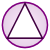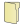Games
Problems
Go Pro!

# Geometry

Pro Problems > Math > Geometry

# Featured Pro ProblemsFind the angle between the diagonals of faces of a cubeAdjust the dimensions of a rectangle until the resulting figure is a squareSeveral points are collinear, and lengths of some segments are given. Can you find the missing length?Angle measures of a triangle, given information about the digits of the anglesFigure out the volume of a wedge of cheese cut from a wheelCalculator may be helpful on this algebra intensive geometry problemIn a triangle the angles meet certain criteria. Can you determine what the angles are?Find the equation of a line that bisects the angle between two other lines# Blogs on This SiteReviews and book lists - books we love!The site administrator fields questions from visitors.Like us on Facebook to get updates about new resources